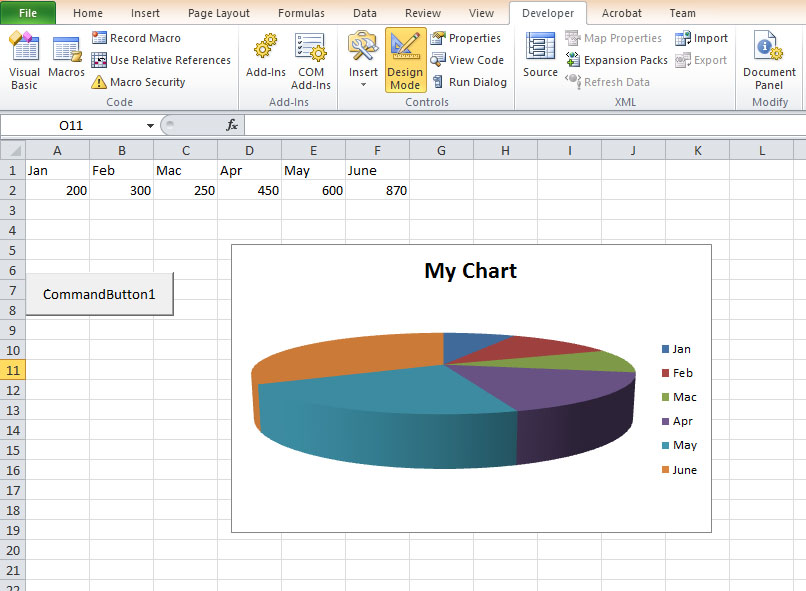# Lesson 24: Creating Charts and Graphs

In Excel VBA , we can write code to create charts and graphs easily based on the data on the spreadsheet. Excel VBA has made charting engine as part of the Shape object. It is also an object by itself. We can create charts on a sheet of their own or embed them into an existing worksheet. The chart sheet is the Chart object whereas the embedded chart is part of the shape collection for the worksheet.

## 24.1 Creating a Pie Chart

To create a pie chart in a spreadsheet, first of all you need to enter a range of data in spreadsheet. After entering the data, you need to name the range by right-clicking the range and clicking define name in the pop-up menu. Name the chart MyChart Now insert a command button and then click it to enter the following code in the Excel VBA editor

```Private Sub CommandButton1_Click()
ActiveSheet.Shapes(1).Top = 10
ActiveSheet.Shapes(1).Left = 10
ActiveChart.ChartType = xl3DPie
ActiveChart.PlotArea.Select
ActiveChart.SetSourceData Source:=Range("MyChart")
ActiveChart.HasTitle = True
ActiveChart.ChartTitle.Text = "My Chart"
End Sub```

### The OutputFigure 24.1: Pie ChartTo separate the pie chart into slices (The technical term is explode), you can add this line to the code:

ActiveChart.SeriesCollection(1).Explosion = 10

As there are five sectors, setting the explosion value to 10 explode all slices. You will get the following chart:

## 24.2 Creating a Bar Graph

To draw a bar graph, you just need to change the ChartType to xl3DColumn.

```Private Sub CommandButton2_Click()
ActiveSheet.Shapes(1).Top = 10
ActiveSheet.Shapes(1).Left = 10
ActiveChart.ChartType = xl3DColumn
ActiveChart.PlotArea.Select
ActiveChart.SetSourceData Source:=Range("MyChart")
ActiveChart.HasTitle = True
ActiveChart.ChartTitle.Text = "My Chart"
End Sub```

Excel VBA has built-in parameters to define the types of charts that can be drawn on a spreadsheet. You can refer to the parameters as the ChartType properties. The list of common properties for ChartType are listed below:

Property Chart Type
xlArea Area Chart
xlBar Bar Chart
xlColumn Column Chart
xlLine Line Chart
xlPie Pie Chart
xlXYScatter XY Scatter Chart
xl3DArea 3D Area Chart
xl3DBar 3D Bar Chart
xl3DColumn 3D Column Chart
Xl3DLine 3D Line Chart

For example, if you change the ChartType property to xlLine using the code

`ActiveChart.ChartType = xlLine`

you can get a line graph as follow: